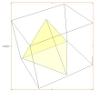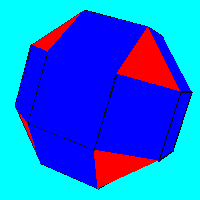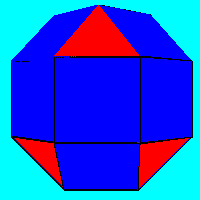#### You may also like### A Mean Tetrahedron

Can you number the vertices, edges and faces of a tetrahedron so that the number on each edge is the mean of the numbers on the adjacent vertices and the mean of the numbers on the adjacent faces?### Icosian Game

This problem is about investigating whether it is possible to start at one vertex of a platonic solid and visit every other vertex once only returning to the vertex you started at.### Triangles to Tetrahedra

Imagine you have an unlimited number of four types of triangle. How many different tetrahedra can you make?

# Rhombicubocts

##### Age 11 to 14Challenge LevelRhombicuboctahedron Pseudo-rhombicuboctahedron

Each of these solids is made up with 3 squares and a triangle around each vertex. Each has a total of 18 square faces and 8 faces that are equilateral triangles. Each has a band of 8 squares around the 'equator' and two square faces at the top and bottom (parallel to the equator) containing the 'north and south poles' at their centres. Draw the net for making each of the shapes and make the models for yourself either with card or a plastic constriction kit. How many faces, edges and vertices does each solid have? How many planes of symmetry and how many axes of rotational symmetry?

The solid on the left is one of the classical semi-regular or Archimedean solids but the one on the right was almost entirely ignored until it was made known by JCP Miller in the 1930s. Perhaps people thought the two were the same - can you describe the differences?